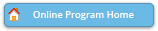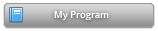#### Abstract Details

 Activity Number: 254 - Contributed Poster Presentations: Section on Bayesian Statistical Science Type: Contributed Date/Time: Monday, July 29, 2019 : 2:00 PM to 3:50 PM Sponsor: Section on Bayesian Statistical Science Abstract #304582 Title: Estimating the Parameters of Circles and Ellipses Using Orthogonal Distance Regression and Bayesian Errors-In-Variables Regression Author(s): Jolene Splett* and Felix Jimenez and Amanda Koepke Companies: National Institute of Standards and Technology and University of Colorado, NIST and National Institute of Standards and Technology Keywords: Bayesian statistics; errors-in-variables regression; orthogonal distance regression; circle fitting; ellipse fitting Abstract: In ordinary least-squares regression, independent variables are assumed to be known without error. However, in many real-life situations this assumption is not valid. Both orthogonal distance regression and Bayesian errors-in-variables regression can be used to estimate model parameters when there are errors in the dependent and independent variables. To illustrate the use of the maximum-likelihood and Bayesian approaches, we use both methods to estimate the parameters of a circle. The data used for circle fitting were taken from the cross section of an optical fiber. The shape of optical fibers is important when joining two fibers, so accurate dimensional measurements are critical to minimizing coupling loss. We then compare the results of the two techniques when fitting an ellipse to simulated data. Circle and ellipse fitting using maximum-likelihood methods have been well documented; however, Bayesian methods for these tasks are less developed. As expected, we found that the Bayesian approach for circle fitting is more intuitive and easier to implement than the maximum-likelihood approach, but generalizing the Bayesian approach to ellipse fitting was surprisingly difficult.

Authors who are presenting talks have a * after their name.